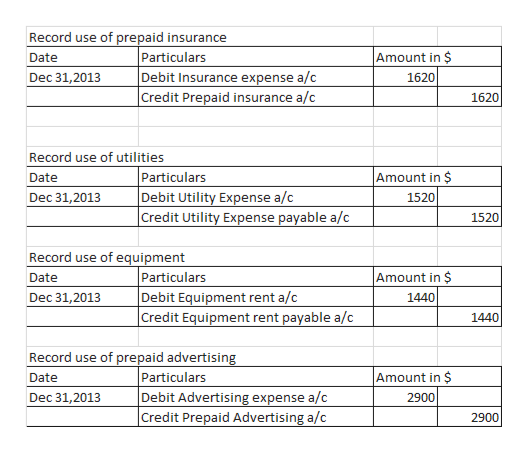Question
125 views

Required:

 Hide

1.  Prepare adjusting entries at December 31 for these four activities.

 Dec. 31

 (Record use of prepaid insurance)

 Dec. 31

 (Record use of utilities)

 Dec. 31

 (Record use of equipment)

 Dec. 31

2.  Conceptual Connection: What would be the effect on expenses if the adjusting entries were not made?

 a. SelectExpenses would be overstated and assets would be overstated, net income and stockholders' equity would be overstated.Expenses would be overstated and assets would be understated, net income and stockholders' equity would be overstated.Expenses would be overstated and liabilities would be overstated, net income and stockholders' equity would be overstated.Expenses would be overstated and liabilities would be understated, net income and stockholders' equity would be overstated.Expenses would be understated and liabilities would be overstated, net income and stockholders' equity would be overstated.Expenses would be understated and liabilities would be understated, net income and stockholders' equity would be overstated.Expenses would be understated and assets would be overstated, net income and stockholders' equity would be overstated.Expenses would be understated and assets would be understated, net income and stockholders' equity would be overstated.Correct 1 of Item 2 b. SelectExpenses would be overstated and assets would be overstated, net income and stockholders' equity would be overstated.Expenses would be overstated and assets would be understated, net income and stockholders' equity would be overstated.Expenses would be overstated and liabilities would be overstated, net income and stockholders' equity would be overstated.Expenses would be overstated and liabilities would be understated, net income and stockholders' equity would be overstated.Expenses would be understated and liabilities would be overstated, net income and stockholders' equity would be overstated.Expenses would be understated and liabilities would be understated, net income and stockholders' equity would be overstated.Expenses would be understated and assets would be overstated, net income and stockholders' equity would be overstated.Expenses would be understated and assets would be understated, net income and stockholders' equity would be overstated.Correct 2 of Item 2 c. SelectExpenses would be overstated and assets would be overstated, net income and stockholders' equity would be overstated.Expenses would be overstated and assets would be understated, net income and stockholders' equity would be overstated.Expenses would be overstated and liabilities would be overstated, net income and stockholders' equity would be overstated.Expenses would be overstated and liabilities would be understated, net income and stockholders' equity would be overstated.Expenses would be understated and liabilities would be overstated, net income and stockholders' equity would be overstated.Expenses would be understated and liabilities would be understated, net income and stockholders' equity would be overstated.Expenses would be understated and assets would be overstated, net income and stockholders' equity would be overstated.Expenses would be understated and assets would be understated, net income and stockholders' equity would be overstated.Correct 3 of Item 2 d. SelectExpenses would be overstated and assets would be overstated, net income and stockholders' equity would be overstated.Expenses would be overstated and assets would be understated, net income and stockholders' equity would be overstated.Expenses would be overstated and liabilities would be overstated, net income and stockholders' equity would be overstated.Expenses would be overstated and liabilities would be understated, net income and stockholders' equity would be overstated.Expenses would be understated and liabilities would be overstated, net income and stockholders' equity would be overstated.Expenses would be understated and liabilities would be understated, net income and stockholders' equity would be overstated.Expenses would be understated and assets would be overstated, net income and stockholders' equity would be overstated.Expenses would be understated and assets would be understated, net income and stockholders' equity would be overstated.Correct 4 of Item 2
Cumulative effect on expenses: SelectOverstatedUnderstatedCorrect 5 of Item 2by \$

check_circle

Step 1

Attached are the adjusting entries to be posted on Dec 31 for transactions mentioned in the question-

Amount for prepaid Insurance entry has been calculated as-
= \$4860/12 x 4mnths= \$1,620
(for Sep to dec i.e. 4mnths out of the...help_outlineImage TranscriptioncloseRecord use of prepaid insurance Particulars Debit Insurance expense a/c Credit Prepaid insurance a/c Amount in \$ Date Dec 31,2013 1620 1620 Record use of utilities Particulars Debit Utility Expense a/c Credit Utility Expense payable a/c Amount in \$ Date Dec 31,2013 1520 1520 Record use of equipment Particulars Debit Equipment rent a/c |Credit Equipment rent payable a/c Amount in \$ Date Dec 31,2013 1440 1440 Record use of prepaid advertising Particulars Debit Advertising expense a/c Credit Prepaid Advertising a/c Amount in \$ Date Dec 31,2013 2900 2900 fullscreen

### Want to see the full answer?

See Solution

#### Want to see this answer and more?

Solutions are written by subject experts who are available 24/7. Questions are typically answered within 1 hour.*

See Solution
*Response times may vary by subject and question.
Tagged in